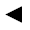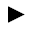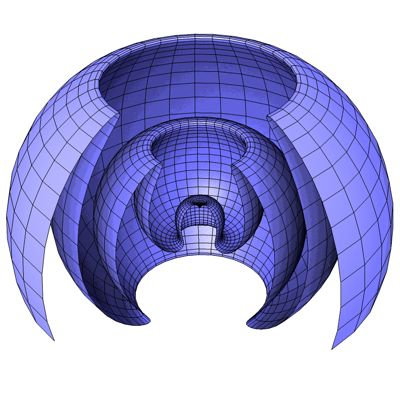[back]

## 3.59. wave ball

[next]The wave sphere [ 9 ] is represented by the following equations.

 x = u cos(cos(u)) cos(v) 3-196 y = u cos(cos(u)) sin(v) 3-197 z = u sin(cos(u)) 3-198

To represent the area, the two parameters u and v can assume the following values ​​(domain of definition), for example.

 u is an element from the set of numbers [0, 14.5] v is an element of the number set [0, 2 pi]

The domain of definition can be changed in the plugin.Fig. 63

Fig. 63 shows the sphere cut open so that the structure can be seen better.